# 01-python中一切皆对象

## python一切皆对象

Python中一切皆对象，在静态语言中，Java也是面向对象编程，Python要比Java的面向对象编程更加彻底。元类编程以及猴子补丁都是用一切皆对象编程出来的。

### 1、函数和类也是对象，是Python的一等公民

1.1、赋值给一个变量

def ask(name=""):
print(name)

my_func("lishuntao")

class Person:
def __init__(self):
print("lishuntao")

my_class = Person     #类对象赋值给变量，然后我们对变量的操作就是对类的操作my_class()

1.2、可以添加到集合对象中

def ask(name="lishuntao"):
print(name)

class Person:
def __init__(self):
print("lishuntao001")

obj_list = []
obj_list.append(Person)
for obj in obj_list:
print(obj())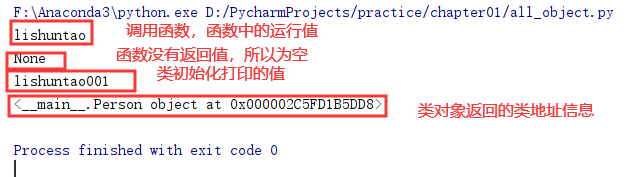1.3、可以作为参数传递给函数

def ask(name="lishuntao"):
print(name)

def print_type(item):
print(type(item))

print(print_type(ask))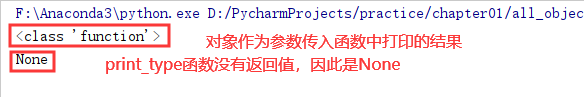1.4、当做函数的返回值

def ask(name="lishuntao"):
print(name)

def decorator_func():
print("start")

my_func = decorator_func()
my_func("Intersting")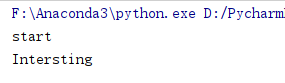### 2、type、object和class之间的关系

a = 11
b = "abc"
c = [1,2]
d = {"a":2}
class Students():
pass

class MyStudents(Students):
pass

stu = Students()
print(type(d))  # <class 'dict'>
print(type(dict))  # <class 'type'>
#                                                   **：type==>list==>c
print(type(a))  #<class 'int'>                      **：type==>dict==>d
print(type(int))  #<class 'type'>                   **：type==>int==>a
print(type(b))   #<class 'str'>                     **：type==>str==>b
print(type(str))  #<class 'type'>                   **：type==>Students==>object
print(type(stu)) #<class '__main__.Students'>       **：type==>type==>MyStudents==>Students==>object
print(type(Students))  #<class 'type'>              **:type类的基类是object，object的基类是空，object的类型是type类，由此可以总结出他们之间的关系
print(int.__bases__) #(<class 'object'>,)
print(str.__bases__) #(<class 'object'>,)
print(Students.__bases__) #(<class 'object'>,)
print(MyStudents.__bases__)#(<class '__main__.Students'>,)
print(type.__bases__)#(<class 'object'>,)
print(object.__bases__)#()
print(type(object))#<class 'type'>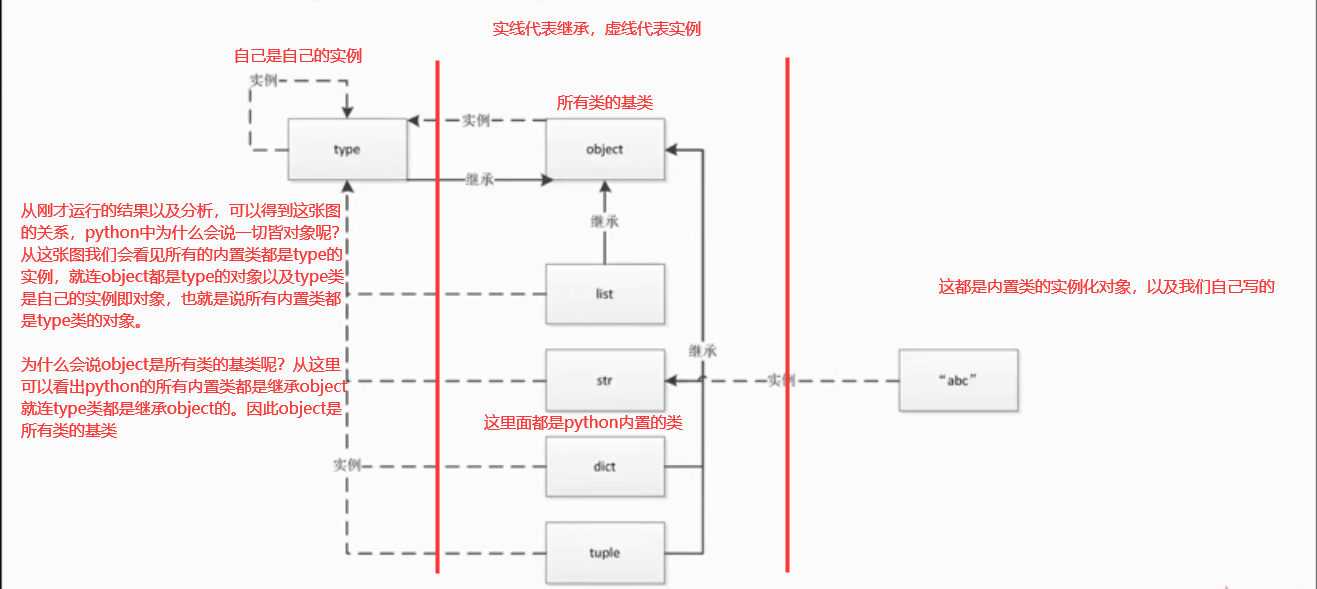### 3、Python中常见内置类型

3.1、对象的三个特征

身份：什么是身份呢？可以理解成对象在内存中的地址。（可以用id函数来查看对象的地址）

类型：对象都是有类型的。（例如：int型，str型）

3.2、类型：Python中有哪些类型

3.2.1、None类型（全局只有一个）：

Python解释器运行的时候，Python会用None这个类型声明一个None的对象。（例如：变量a、b都被赋值一个None对象，然后会发现变量都指向一个None对象（地址可以看出，例如下图））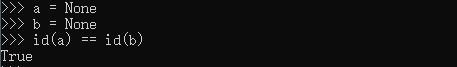3.2.2、数值类型：

int类型、float类型、complex(复数)类型、bool类型

3.2.3、迭代类型：

迭代类型用for循环进行遍历。（在后边的迭代器和生成器学习会介绍）

3.2.4、序列类型：

常见的有list、tuple、str、array、range、bytes，bytearray，memoryview（二进制序列）等。

3.2.5、映射类型(dict)：

常用的字典，是一个映射类型。映射类型就是有keymap的。

3.2.6、集合：

set、frozenset（不可以修改的set

3.2.7、上下文管理类型(with)

with语句

3.2.8其他类型：

模块类型（from，import）、class和实例、函数类型、方法类型（方法和函数是有区别的。方法是类中的函数）、代码类型（代码本身也会被Python解释器变为一个对象类型）、object对象、type类型、ellipsis类型（省略号类型）、notimplemented类型

posted @ 2019-11-28 14:39  一知.半解  阅读(...)  评论(...编辑  收藏